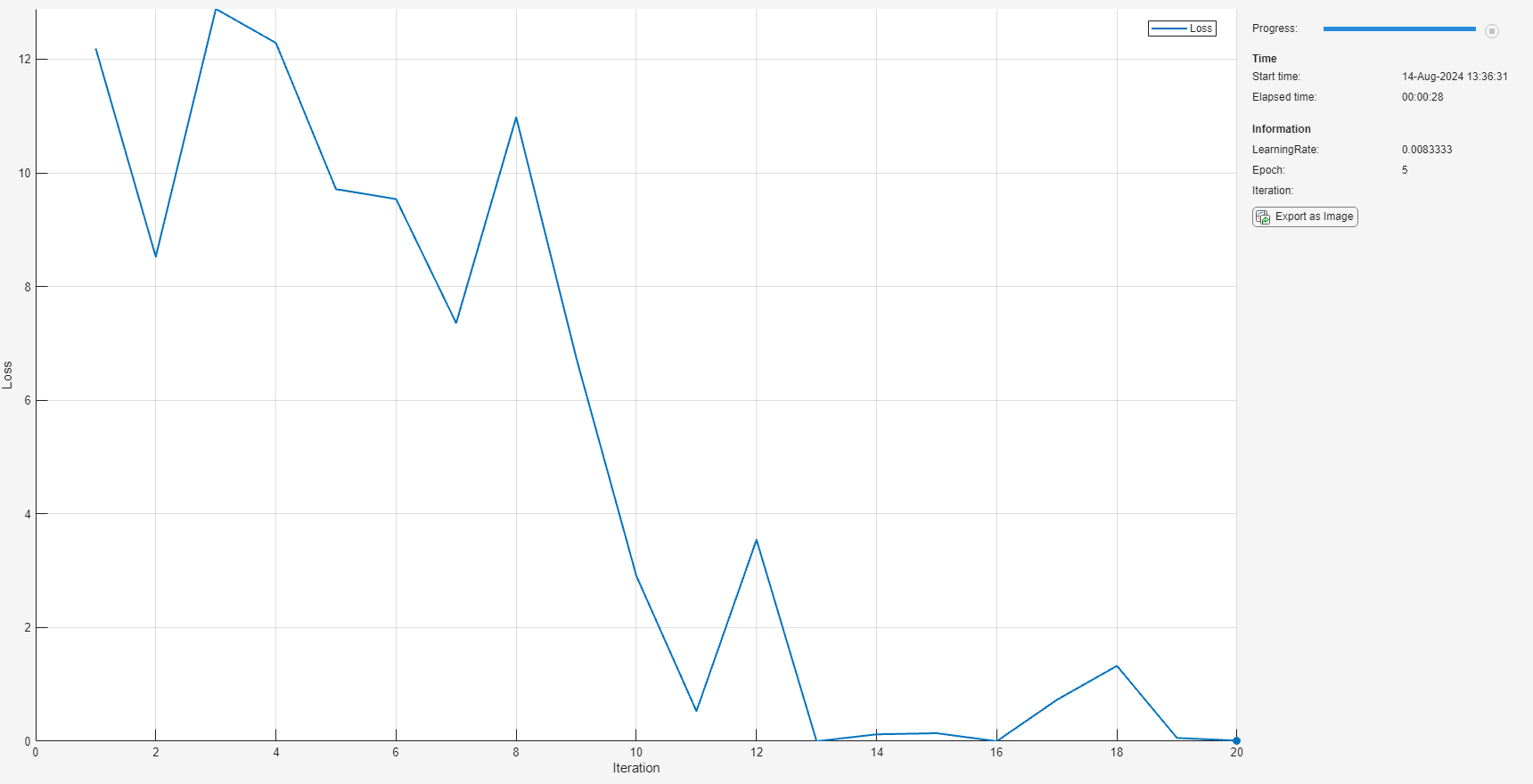# unfreezeParameters

Convert nonlearnable network parameters in `ONNXParameters` to learnable

## Syntax

``params = unfreezeParameters(params,names)``

## Description

example

````params = unfreezeParameters(params,names)` unfreezes the network parameters specified by `names` in the `ONNXParameters` object `params`. The function moves the specified parameters from `params.Nonlearnables` in the input argument `params` to `params.Learnables` in the output argument `params`.```

## Examples

collapse all

Import the `squeezenet` convolution neural network as a function and fine-tune the pretrained network with transfer learning to perform classification on a new collection of images.

This example uses several helper functions. To view the code for these functions, see Helper Functions.

Unzip and load the new images as an image datastore. `imageDatastore` automatically labels the images based on folder names and stores the data as an `ImageDatastore` object. An image datastore enables you to store large image data, including data that does not fit in memory, and efficiently read batches of images during training of a convolutional neural network. Specify the mini-batch size.

```unzip('MerchData.zip'); miniBatchSize = 8; imds = imageDatastore('MerchData', ... 'IncludeSubfolders',true, ... 'LabelSource','foldernames',... 'ReadSize', miniBatchSize);```

This data set is small, containing 75 training images. Display some sample images.

```numImages = numel(imds.Labels); idx = randperm(numImages,16); figure for i = 1:16 subplot(4,4,i) I = readimage(imds,idx(i)); imshow(I) end```Extract the training set and one-hot encode the categorical classification labels.

```XTrain = readall(imds); XTrain = single(cat(4,XTrain{:})); YTrain_categ = categorical(imds.Labels); YTrain = onehotencode(YTrain_categ,2)';```

Determine the number of classes in the data.

```classes = categories(YTrain_categ); numClasses = numel(classes)```
```numClasses = 5 ```

`squeezenet` is a convolutional neural network that is trained on more than a million images from the ImageNet database. As a result, the network has learned rich feature representations for a wide range of images. The network can classify images into 1000 object categories, such as keyboard, mouse, pencil, and many animals.

Import the pretrained `squeezenet` network as a function.

```squeezenetONNX() params = importONNXFunction('squeezenet.onnx','squeezenetFcn')```
```A function containing the imported ONNX network has been saved to the file squeezenetFcn.m. To learn how to use this function, type: help squeezenetFcn. ```
```params = ONNXParameters with properties: Learnables: [1×1 struct] Nonlearnables: [1×1 struct] State: [1×1 struct] NumDimensions: [1×1 struct] NetworkFunctionName: 'squeezenetFcn' ```

`params` is an `ONNXParameters` object that contains the network parameters. `squeezenetFcn` is a model function that contains the network architecture. `importONNXFunction` saves `squeezenetFcn` in the current folder.

Calculate the classification accuracy of the pretrained network on the new training set.

```accuracyBeforeTraining = getNetworkAccuracy(XTrain,YTrain,params); fprintf('%.2f accuracy before transfer learning\n',accuracyBeforeTraining);```
```0.01 accuracy before transfer learning ```

The accuracy is very low.

Display the learnable parameters of the network by typing `params.Learnables`. These parameters, such as the weights (`W`) and bias (`B`) of convolution and fully connected layers, are updated by the network during training. Nonlearnable parameters remain constant during training.

The last two learnable parameters of the pretrained network are configured for 1000 classes.

`conv10_W: [1×1×512×1000 dlarray]`

`conv10_B: [1000×1 dlarray]`

The parameters `conv10_W` and `conv10_B` must be fine-tuned for the new classification problem. Transfer the parameters to classify five classes by initializing the parameters.

```params.Learnables.conv10_W = rand(1,1,512,5); params.Learnables.conv10_B = rand(5,1);```

Freeze all the parameters of the network to convert them to nonlearnable parameters. Because you do not need to compute the gradients of the frozen layers, freezing the weights of many initial layers can significantly speed up network training.

`params = freezeParameters(params,'all');`

Unfreeze the last two parameters of the network to convert them to learnable parameters.

```params = unfreezeParameters(params,'conv10_W'); params = unfreezeParameters(params,'conv10_B');```

Now the network is ready for training. Initialize the training progress plot.

```plots = "training-progress"; if plots == "training-progress" figure lineLossTrain = animatedline; xlabel("Iteration") ylabel("Loss") end```

Specify the training options.

```velocity = []; numEpochs = 5; miniBatchSize = 16; numObservations = size(YTrain,2); numIterationsPerEpoch = floor(numObservations./miniBatchSize); initialLearnRate = 0.01; momentum = 0.9; decay = 0.01;```

Train the network.

```iteration = 0; start = tic; executionEnvironment = "cpu"; % Change to "gpu" to train on a GPU. % Loop over epochs. for epoch = 1:numEpochs % Shuffle data. idx = randperm(numObservations); XTrain = XTrain(:,:,:,idx); YTrain = YTrain(:,idx); % Loop over mini-batches. for i = 1:numIterationsPerEpoch iteration = iteration + 1; % Read mini-batch of data. idx = (i-1)*miniBatchSize+1:i*miniBatchSize; X = XTrain(:,:,:,idx); Y = YTrain(:,idx); % If training on a GPU, then convert data to gpuArray. if (executionEnvironment == "auto" && canUseGPU) || executionEnvironment == "gpu" X = gpuArray(X); end % Evaluate the model gradients and loss using dlfeval and the % modelGradients function. [gradients,loss,state] = dlfeval(@modelGradients,X,Y,params); params.State = state; % Determine the learning rate for the time-based decay learning rate schedule. learnRate = initialLearnRate/(1 + decay*iteration); % Update the network parameters using the SGDM optimizer. [params.Learnables,velocity] = sgdmupdate(params.Learnables,gradients,velocity); % Display the training progress. if plots == "training-progress" D = duration(0,0,toc(start),'Format','hh:mm:ss'); addpoints(lineLossTrain,iteration,double(gather(extractdata(loss)))) title("Epoch: " + epoch + ", Elapsed: " + string(D)) drawnow end end end```Calculate the classification accuracy of the network after fine-tuning.

```accuracyAfterTraining = getNetworkAccuracy(XTrain,YTrain,params); fprintf('%.2f accuracy after transfer learning\n',accuracyAfterTraining);```
```1.00 accuracy after transfer learning ```

Helper Functions

This section provides the code of the helper functions used in this example.

The `getNetworkAccuracy` function evaluates the network performance by calculating the classification accuracy.

```function accuracy = getNetworkAccuracy(X,Y,onnxParams) N = size(X,4); Ypred = squeezenetFcn(X,onnxParams,'Training',false); [~,YIdx] = max(Y,[],1); [~,YpredIdx] = max(Ypred,[],1); numIncorrect = sum(abs(YIdx-YpredIdx) > 0); accuracy = 1 - numIncorrect/N; end```

The `modelGradients` function calculates the loss and gradients.

```function [grad, loss, state] = modelGradients(X,Y,onnxParams) [y,state] = squeezenetFcn(X,onnxParams,'Training',true); loss = crossentropy(y,Y,'DataFormat','CB'); grad = dlgradient(loss,onnxParams.Learnables); end```

The `squeezenetONNX` function generates an ONNX model of the `squeezenet` network.

```function squeezenetONNX() exportONNXNetwork(squeezenet,'squeezenet.onnx'); end ```

## Input Arguments

collapse all

Network parameters, specified as an `ONNXParameters` object. `params` contains the network parameters of the imported ONNX™ model.

Names of the parameters to unfreeze, specified as `'all'` or a string array. Unfreeze all nonlearnable parameters by setting `names` to `'all'`. Unfreeze `k` nonlearnable parameters by defining the parameter names in the 1-by-`k` string array `names`.

Example: `["gpu_0_sl_pred_b_0", "gpu_0_sl_pred_w_0"]`

Data Types: `char` | `string`

## Output Arguments

collapse all

Network parameters, returned as an `ONNXParameters` object. `params` contains the network parameters updated by `unfreezeParameters`.